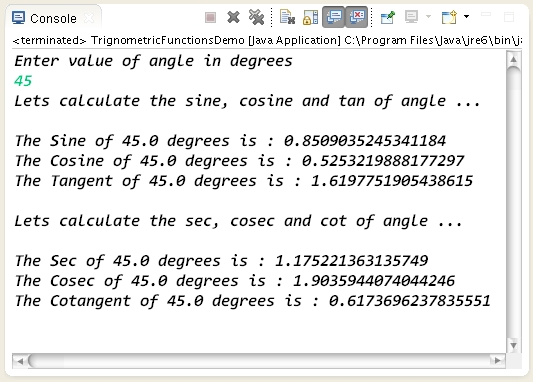### How to calculate Trigonometric functions values in Java ?

 Tweet Program to demonstrate how to calculate Trigonometric functions values in Java ```package com.hubberspot.code; import java.util.Scanner; public class TrignometricFunctionsDemo { public static void main(String[] args) { // Create a Scanner object which will read // values from the console which user enters Scanner scanner = new Scanner(System.in); // Getting input from user from the console System.out.println("Enter value of angle in degrees "); // Calling nextDouble method of scanner for // taking a double value from user and storing // it in degrees variable double degrees = scanner.nextDouble(); System.out.println("Lets calculate the sine, cosine and tan of angle ..."); // In order to calculate sine , cosine and tan of angle we // use the Math class three static methods by name as : // 1. Math.sin(a) -- Sine of a // 2. Math.cos(a) -- Cosine of a // 3. Math.tan(a) -- Tangent of a double sineOfAngle = Math.sin(degrees); double cosOfAngle = Math.cos(degrees); double tanOfAngle = Math.tan(degrees); System.out.println(); System.out.println("The Sine of " + degrees + " degrees is : " + sineOfAngle); System.out.println("The Cosine of " + degrees + " degrees is : " + cosOfAngle); System.out.println("The Tangent of " + degrees + " degrees is : " + tanOfAngle); System.out.println(); System.out.println("Lets calculate the sec, cosec and cot of angle ..."); // In order to calculate sec, cosec and cot of angle we // just inverse the value of sin , cos and tan calculated above : // 4. Sec of a -- 1 / Sine of a // 5. Cosec of a -- 1/ Cosine of a // 6. Cot of a -- 1 / Tangent of a double secOfAngle = 1 / Math.sin(degrees); double cosecOfAngle = 1 / Math.cos(degrees); double cotOfAngle = 1 / Math.tan(degrees); System.out.println("\nThe Sec of " + degrees + " degrees is : " + secOfAngle); System.out.println("The Cosec of " + degrees + " degrees is : " + cosecOfAngle); System.out.println("The Cotangent of " + degrees + " degrees is : " + cotOfAngle); } } ``` Output of the program :Program to demonstrate how to calculate Trigonometric functions values in Java

```package com.hubberspot.code;

import java.util.Scanner;

public class TrignometricFunctionsDemo {

public static void main(String[] args) {

// Create a Scanner object which will read
// values from the console which user enters
Scanner scanner = new Scanner(System.in);

// Getting input from user from the console
System.out.println("Enter value of angle in degrees ");

// Calling nextDouble method of scanner for
// taking a double value from user and storing
// it in degrees variable
double degrees = scanner.nextDouble();

System.out.println("Lets calculate the sine, cosine and tan of angle ...");
// In order to calculate sine , cosine and tan of angle we
// use the Math class three static methods by name as :
// 1. Math.sin(a) -- Sine of a
// 2. Math.cos(a) -- Cosine of a
// 3. Math.tan(a) -- Tangent of a

double sineOfAngle = Math.sin(degrees);
double cosOfAngle = Math.cos(degrees);
double tanOfAngle = Math.tan(degrees);

System.out.println();
System.out.println("The Sine of " + degrees + " degrees is : "
+ sineOfAngle);
System.out.println("The Cosine of " + degrees + " degrees is : "
+ cosOfAngle);
System.out.println("The Tangent of " + degrees + " degrees is : "
+ tanOfAngle);

System.out.println();
System.out.println("Lets calculate the sec, cosec and cot of angle ...");
// In order to calculate sec, cosec and cot of angle we
// just inverse the value of sin , cos and tan calculated above :
// 4. Sec of a -- 1 / Sine of a
// 5. Cosec of a  -- 1/ Cosine of a
// 6. Cot of a  -- 1 / Tangent of a

double secOfAngle = 1 / Math.sin(degrees);
double cosecOfAngle = 1 / Math.cos(degrees);
double cotOfAngle = 1 / Math.tan(degrees);

System.out.println("\nThe Sec of " + degrees + " degrees is : "
+ secOfAngle);
System.out.println("The Cosec of " + degrees + " degrees is : "
+ cosecOfAngle);
System.out.println("The Cotangent of " + degrees + " degrees is : "
+ cotOfAngle);

}

}

```

Output of the program :

Please Give Us Your 1 Minute In Sharing This Post!
SOCIALIZE IT →